Updated in 2563 : on2020 :3 :10 :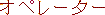Operator ;

equal; match;Radical102;

division operator;Radical286;

Following table contains commonly use operators; Notice that Visual Basic, using more operators thanJava; HARDWARE related i.e.calculator,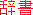dictionary,translator,GPS, Idea Processor, ... , operators are also included in thistable; Being a significant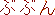part ... ;Artificial Intelligence (Calculator Key) e.g.IFF ((left)Operator (right)), kuru kuruWHILE, higher (e.g. bigger) number is Val (value, e.g. constant), lower (e.g. smaller) number is Ref (reference, e.g. variable); Val and Ref can be vice versa;IFF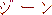genecodes, (neitherhyper, nor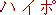hypo)is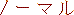normal;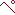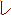operatoradding operator + ( addition operator ) addition operator ( + ) algebraic operator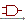And ( logical ) operator And Also operator arithmetic ( or mathematical ) operator s arithmetic operator assignment operator = ( assignment or equal to operator ) assignment or equal to operator ( = ) binary operator Boolean operator cable operator comparison ( or relational operator s complement operator concatenation operator decimal operator / ( division operator ) division operator division operator ( / ) ^ ( exponential operator ) exponential operator exponential operator ( * * ) exponential operator ( ^ ) equal to or assignment operator ( = ) > ( greater than operator ) > = ( greater than or equal to operator ) greater than operator ( > ) greater than or equal to operator ( > = ) < ( less than operator ) < = ( less than or equal to operator ) less than operator ( < ) less than or equal to operator ( < = ) list operator local operator logical operator logical operator s match ( i n g ) operator mathematical ( or arithmetic ) operator s Mod ( remainder division operator ) monadic operator * ( multiplication operator ) multiplication operator ( * ) non - operator mode < > ( not equal to operator )Not ( logical operator )not equal to operator ( < > ) ( operator ) console operatorOr ( logical operator ) Or Else operator range operator ( . . ) relation relational operator - ( subtraction ) operator substitution operator subtraction operator ( - ) & ( string concatenation operator ) string concatenation operator ( & ) system operator ( s y s o p ) type conversion operator unary operator X or ( logical operator ) + operator , for string concatenation , . ( dot operator ) , = = ( equality operator ) , equality operator ( = = ) ,

in common, "<" i.e. less than, "=" i.e. equal to, ">" i.e. bigger than;
Left Angle Bracket < 's ASCII code number is 60; Equal = 's ASCII code number is 61; Right Angle Bracket > 's ASCII code number is 62;Radicals, also see: counter;

mathematical operators, also see: AI FONT;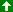Up